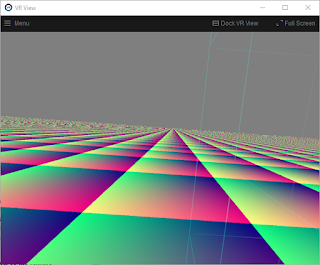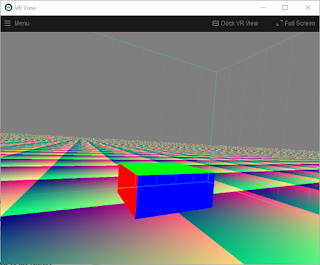## Wednesday, May 13, 2020

### Infinite Plane Rendering 2: Texturing and depth buffer

This is the second post about rendering infinite planes in 3D computer graphics. Last time we got as far as rendering a uniform brown plane. This time we will add texturing and correct use of the Z/depth buffer."Brown plane" example from the previous post.

### Simplifying the math

Now that I'm looking at all this again, I see that we can simplify the plane math somewhat. Sorry I'm now going to change the variable names to match the plane ray tracing derivation in the OpenGL Super Bible. By the way, we aren't actually doing ray tracing in this infinite plane project, but rather simply "ray casting".

Remember the implicit plane equation from last time:

Ax + By + Cz + Dw = 0  (1b)

Where:

• (A B C) is a vector normal to the plane,
• (x y z) is any point in the plane, and
• D is the signed distance from the plane to the origin

We can rewrite that in vector form:

P·N + d = 0    (1c)

Where:

• N is a vector perpendicular to the plane
• P is any point on the plane, and
• d is the signed distance of the plane from the origin
That dot represents the dot product or inner product of two vectors.

We will combine the plane equation above with the ray equation below:

P = O + tD    (3)

Where:

• P is any point along the ray,
• O is the origin of the ray (i.e. the camera/viewer location),
• D is the direction of the view ray, and
• t is the scalar ray parameter.
Plug (3) into (1c) to get:

(O + tD)·N + d = 0

now solve for t:

t = -(O·N + d)/(D·N)

and plug that back into the ray equation to get I, the intersection between the plane and the view ray:

I = O - D(O·N + d)/(D·N)

Now we can simplify this further: If we solve for the intersection of the plane and the view ray in view/camera space, then the view ray origin O, the position of the camera/eye, is all zeros (0, 0, 0), and the intersection point equation reduces to:

I = -dD/(D·N)  (4)

That's pretty simple.

In our vertex shader, we compute the intersection point and pass it into the fragment shader as a homogeneous coordinate, with the denominator in the homogeneous w coordinate, as we discussed last time. A GLSL vertex shader code fragment is shown below:

point = vec4(
-d * D.xyz,  // xyz, numerator
dot(D.xyz, N.xyz)  // w, denominator

);

### Texturing the plane

That solid brown plane from the previous post could be hiding all sorts of errors. By adding texturing to the plane, we can visually distinguish different parts of the plane, so it becomes much easier to carefully verify correct rendering from inside the VR headset.

There are 4 different types of texturing we could apply to our plane:

1. Solid color rendering, like we did in the previous brown plane example.
2. Ordinary 2D image texture rendering, where an image pattern is repeated over the plane.
3. Procedural texture rendering, where a computed texture is applied in the fragment shader.
4. Spherical image texture rendering, using a 360 image. This way we can paint the whole plane with one single image. That's a great way to combine the special "whole universe" coverage of spherical panoramas, with the "half universe" coverage of infinite planes. We will get to this final case in a future post.
For now we will start with the simplest of procedural textures: a color version of the texture coordinates themselves. This helps us to debug texturing, and establishes the basis for other more advanced texturing schemes.A simple procedural texture tiling this infinite plane.
Now with the texturing in place, we can inspect the plane more carefully in VR, to help debug any rendering defects. For now it's (mostly) looking pretty good.

The grainy junk near the horizon is there because we have no texture filtering. Texture filtering for procedural textures like this is an advanced and difficult task. We won't be solving it here, because this is just a waypoint for us on the way to ordinary image-based texturing. There, the filtering techniques available to us will become much more conventional and straightforward.

### Populating the depth buffer

I mentioned in the previous post that our initial brown plane example does not correctly clip through other objects. Let's correct that now.

We can compute the correct depth buffer value for the plane intersection point by first converting the plane/ray intersection point into clip space (Normalized Device Coordinates) and then insert the depth value into the Z-buffer in the fragment shader.

vec4 ndc = projection * point;
...
gl_FragDepth = (ndc.z / ndc.w + 1.0) / 2.0;Notice the top section of a cube mesh being correctly clipped by the infinite plane. The rest of the cube is correctly hidden beneath the surface of the plane. Thanks to gl_FragDepth.
There is one more nuance to using infinite planes with the depth buffer. We need to use a special sort of projection matrix that goes all the way to infinity, i.e. it has no far clip plane. So, for example, two infinite planes at different angles would clip each other correctly all the way to the horizon. To infinity! (but not beyond...)

Shader code for this version is at https://gist.github.com/cmbruns/3c184d303e665ee2e987e4c1c2fe4b56

### Topics for future posts:

• Image-based texturing
• Antialiasing
• Drawing the horizon line
• What happens if I look underneath that plane?
• More efficient imposter geometries for rendering